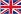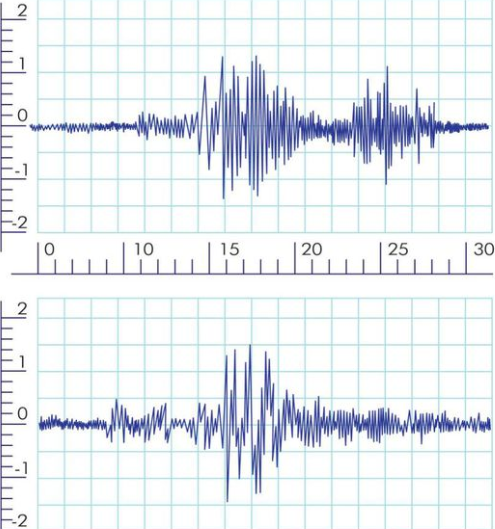  +86-15658151051                          sales@xingultrasonic.comEnglish
Articles Detail
/ / / What's the relationship bewteen ultrasonic amplitude and frequency

# What's the relationship bewteen ultrasonic amplitude and frequency

Views:0     Author:Site Editor     Publish Time: 2019-07-26      Origin:Site

What's the relationship between ultrasonic amplitude and frequency?

Frequency has nothing to do with amplitude.

The number of full vibrations completed by the vibrating mass in one second is called the frequency of the vibration, and its unit is Hertz (Hz). The frequency is also the physical quantity indicating how fast the particle vibrates. The higher the frequency, the faster the vibration. Amplitude is the large value that the physical quantity of vibration can reach, usually expressed in A. It is a physical quantity that represents the range and intensity of vibration.

The relationship between the period and the frequency or its unit relationship is 1 Hz = 1 S ^ ( -1 ) The natural frequency and the natural frequency of the harmonic vibration motion (cycle) is determined by the nature of the vibrating object itself, so it is called the natural frequency (the natural period) ). The frequency of the sound waves determines the pitch of the sound.Frequency and amplitude

In mechanical vibration, the amplitude is the absolute value of the large displacement from the equilibrium position when the object vibrates, and the amplitude is numerically equal to the magnitude of the large displacement. The amplitude is a scalar and the unit is expressed in meters or centimeters. The amplitude describes the magnitude of the vibration amplitude of the object and the strength of the vibration.

In an AC circuit, the current amplitude or voltage amplitude is a large value of the current or voltage change, also called the peak value of the voltage or current. In acoustic vibration, the amplitude is a large value of the difference between the sound pressure and the static pressure. The amplitude of the sound wave is in decibels. The magnitude of the amplitude of the sound wave can determine the intensity of the sound.

The amplitude of the harmonic vibration is constant, which is a constant determined by the initial conditions of the harmonic motion (initial displacement and initial velocity). The energy of the harmonic motion is proportional to the square of the amplitude. Therefore, the square of the amplitude can be used as a sign of the harmonic strength of the resonance. The amplitude of the steady phase of forced vibration is also a constant, and the amplitude of the damped vibration is gradually reduced.